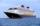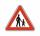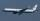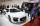# Two cyclists 2

At the same time, two cyclists left the towns A and B at constant speeds. The first one going from town A to town B, and the second one from town B to town A. At one point of the trip they met. After they met, the first cyclist arrived at town B in 36min, the second cyclist arrived at town A in 25min. How long did it take for the first cyclist to reach the meeting point after leaving town A?

Result

t1 =  30 min

#### Solution:Leave us a comment of example and its solution (i.e. if it is still somewhat unclear...):

Showing 0 comments:Be the first to comment!#### To solve this verbal math problem are needed these knowledge from mathematics:

Looking for help with calculating harmonic mean? Looking for a statistical calculator? Looking for help with calculating roots of a quadratic equation? Do you have a linear equation or system of equations and looking for its solution? Or do you have quadratic equation? Do you want to convert length units?

## Next similar examples:

1. The cruise shipThe cruise ship has a speed of 12 km / h at a calm surface. When we sail 45 km along the river and 45 km back, it took us exactly 8 hours. Which (constant) speed of flow of the river?
2. WavelengthCalculate the wavelength of the tone frequency 11 kHz if the sound travels at speeds of 343 m/s.
3. WalkersFrom points A and B simultaneously started against each other two walkers. After meeting both continue to B. Second walker came to B 2 hours before the first walker. It's speed is 2.7 times of speed of the first pedestrian. How many hours went pedestria
4. Water current speed.Two cities along the river are 100 km apart. The powerboat downstream runs for 4 hours, upstream for 10 hours. Determines the river's current speed.
5. Two planesTwo planes flying from airports A and B, 420 km distant against each other. Plane from A took off 15 minutes later and flies at an average speed of 40 km/h higher than the plane from B. Determine the average speed of this two aircraft if you know that it
6. TrainsFrom station 130 km away started passenger train and after 2.2 hours after the express train, which travels 37 km an hour more. Express train finish journey 7 minutes early. Calculate the average speed of this two trains.
7. Speed of carThe car went to a city that was 240 km away. If his speed increased by 8 km/h, it would reach the finish one hour earlier. Determine its original speed.
8. Square root 2If the square root of 3m2 +22 and -x = 0, and x=7, what is m?
9. Evaluation of expressionsIf a2-3a+1=0, find (i)a2+1/a2 (ii) a3+1/a3
10. RootsDetermine the quadratic equation absolute coefficient q, that the equation has a real double root and the root x calculate: ?
11. Ball gameRichard, Denis and Denise together scored 932 goals. Denis scored 4 goals over Denise but Denis scored 24 goals less than Richard. Determine the number of goals for each player.
12. Theorem proveWe want to prove the sentence: If the natural number n is divisible by six, then n is divisible by three. From what assumption we started?
13. EquationEquation ? has one root x1 = 8. Determine the coefficient b and the second root x2.
14. Solve 3Solve quadratic equation: (6n+1) (4n-1) = 3n2
15. DiscriminantDetermine the discriminant of the equation: ?
16. Quadratic equationFind the roots of the quadratic equation: 3x2-4x + (-4) = 0.
17. Linsys2Solve two equations with two unknowns: 400x+120y=147.2 350x+200y=144